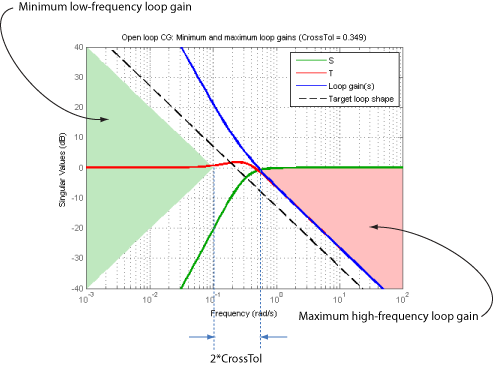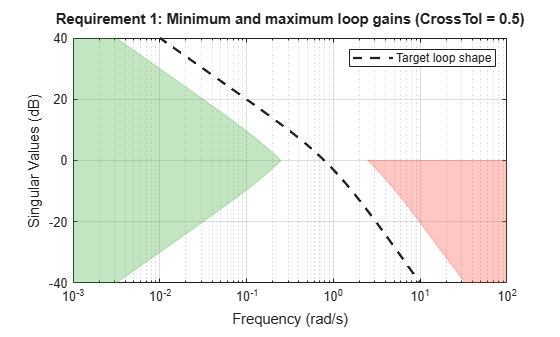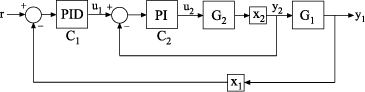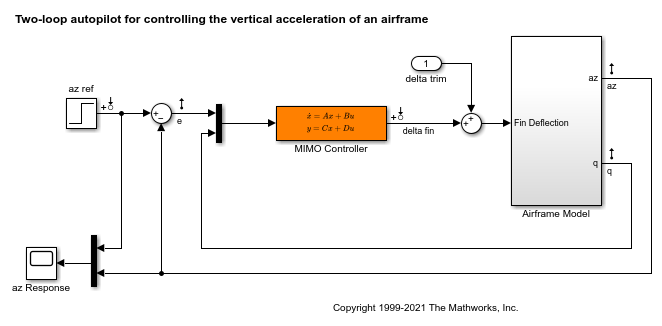TuningGoal.LoopShape class

Package: TuningGoal

Target loop shape for control system tuning

Description

Use TuningGoal.LoopShape to specify a target gain profile (gain as a function of frequency) of an open-loop response. TuningGoal.LoopShape constrains the open-loop, point-to-point response (L) at a specified location in your control system. Use this tuning goal for control system tuning with tuning commands, such as systune or looptune.

When you tune a control system, the target open-loop gain profile is converted into constraints on the inverse sensitivity function inv(S)  = (I + L) and the complementary sensitivity function T = 1–S. These constraints are illustrated for a representative tuned system in the following figure.Where L is much greater than 1, a minimum gain constraint on inv(S) (green shaded region) is equivalent to a minimum gain constraint on L. Similarly, where L is much smaller than 1, a maximum gain constraint on T (red shaded region) is equivalent to a maximum gain constraint on L. The gap between these two constraints is twice the CrossTol parameter, which specifies the frequency band where the loop gain can cross 0 dB.

For multi-input, multi-output (MIMO) control systems, values in the gain profile greater than 1 are interpreted as minimum performance requirements. Such values are lower bounds on the smallest singular value of the open-loop response. Gain profile values less than one are interpreted as minimum roll-off requirements, which are upper bounds on the largest singular value of the open-loop response. For more information about singular values, see sigma.

Use TuningGoal.LoopShape when the loop shape near crossover is simple or well understood (such as integral action). To specify only high gain or low gain constraints in certain frequency bands, use TuningGoal.MinLoopGain and TuningGoal.MaxLoopGain. When you do so, the software determines the best loop shape near crossover.

Construction

Req = TuningGoal.LoopShape(location,loopgain) creates a tuning goal for shaping the open-loop response measured at the specified location. The magnitude of the single-input, single-output (SISO) transfer function loopgain specifies the target open-loop gain profile. You can specify the target gain profile (maximum gain across the I/O pair) as a smooth transfer function or sketch a piecewise error profile using an frd model.

Req = TuningGoal.LoopShape(location,loopgain,crosstol) specifies a tolerance on the location of the crossover frequency. crosstol expresses the tolerance in decades. For example, crosstol = 0.5 allows gain crossovers within half a decade on either side of the target crossover frequency specified by loopgain. When you omit crosstol, the tuning goal uses a default value of 0.1 decades. You can increase crosstol when tuning MIMO control systems. Doing so allows more widely varying crossover frequencies for different loops in the system.

Req = TuningGoal.LoopShape(location,wc) specifies just the target gain crossover frequency. This syntax is equivalent to specifying a pure integrator loop shape, loopgain = wc/s.

Req = TuningGoal.LoopShape(location,wcrange) specifies a range for the target gain crossover frequency. The range is a vector of the form wcrange = [wc1,wc2]. This syntax is equivalent to using the geometric mean sqrt(wc1*wc2) as wc and setting crosstol to the half-width of wcrange in decades. Using a range instead of a single wc value increases the ability of the tuning algorithm to enforce the target loop shape for all loops in a MIMO control system.

Examples

collapse all

Create a target gain profile requirement for the following control system. Specify integral action, gain crossover at 1, and a roll-off requirement of 40 dB/decade.The requirement should apply to the open-loop response measured at the AnalysisPoint block X. Specify a crossover tolerance of 0.5 decades.

LS = frd([100 1 0.0001],[0.01 1 100]);
Req = TuningGoal.LoopShape('X',LS,0.5);

The software converts LS into a smooth function of frequency that approximates the piecewise-specified requirement. Display the requirement using viewGoal.

viewGoal(Req)The green and red regions indicate the bounds for the inverse sensitivity, inv(S) = 1-G*C, and the complementary sensitivity, T = 1-S, respectively. The gap between these regions at 0 dB gain reflects the specified crossover tolerance, which is half a decade to either side of the target loop crossover.

When you use viewGoal(Req,CL) to validate a tuned closed-loop model of this control system, CL, the tuned values of S and T are also plotted.

Create separate loop shape requirements for the inner and outer loops of the following control system.For the inner loop, specify a loop shape with integral action, gain crossover at 1, and a roll-off requirement of 40 dB/decade. Additionally, specify that this loop shape requirement should be enforced with the outer loop open.

LS2 = frd([100 1 0.0001],[0.01 1 100]);
Req2 = TuningGoal.LoopShape('X2',LS2);
Req2.Openings = 'X1';

Specifying 'X2' for the location indicates that Req2 applies to the point-to point, open-loop transfer function at the location X2. Setting Req2.Openings indicates that the loop is opened at the analysis point X1 when Req2 is enforced.

By default, Req2 imposes a stability requirement on the inner loop as well as the loop shape requirement. In some control systems, however, inner-loop stability might not be required, or might be impossible to achieve. In that case, remove the stability requirement from Req2 as follows.

Req2.Stabilize = false;

For the outer loop, specify a loop shape with integral action, gain crossover at 0.1, and a roll-off requirement of 20 dB/decade.

LS1 = frd([10 1 0.01],[0.01 0.1 10]);
Req1 = TuningGoal.LoopShape('X1',LS1);

Specifying 'X1' for the location indicates that Req1 applies to the point-to point, open-loop transfer function at the location X1. You do not have to set Req1.Openings because this loop shape is enforced with the inner loop closed.

You might want to tune the control system with both loop shaping requirements Req1 and Req2. To do so, use both requirements as inputs to the tuning command. For example, suppose CL0 is a tunable genss model of the closed-loop control system. In that case, use [CL,fSoft] = systune(CL0,[Req1,Req2]) to tune the control system to both requirements.

Create a loop-shape requirement for the feedback loop on 'q' in the Simulink model rct_airframe2. Specify that the loop-shape requirement is enforced with the 'az' loop open.

Open the model.

open_system('rct_airframe2')Create a loop shape requirement that enforces integral action with a crossover a 2 rad/s for the 'q' loop. This loop shape corresponds to a loop shape of 2/_s_.

s = tf('s');
shape = 2/s;
Req = TuningGoal.LoopShape('q',shape);

Specify the location at which to open an additional loop when enforcing the requirement.

Req.Openings = 'az';

To use this requirement to tune the Simulink model, create an slTuner interface to the model. Identify the block to tune in the interface.

ST0 = slTuner('rct_airframe2','MIMO Controller');

Designate both az and q as analysis points in the slTuner interface.

This command makes q available as an analysis location. It also allows the tuning requirement to be enforced with the loop open at az.

You can now tune the model using Req and any other tuning requirements. For example:

[ST,fSoft] = systune(ST0,Req);
Final: Soft = 0.845, Hard = -Inf, Iterations = 51

Create a tuning requirement specifying that the open-loop response of loop identified by 'X' cross unity gain between 50 and 100 rad/s.

Req = TuningGoal.LoopShape('X',[50,100]);

Examine the resulting requirement to see the target loop shape.

viewGoal(Req)The plot shows that the requirement specifies an integral loop shape, with crossover around 70 rad/s, the geometrical mean of the range [50,100]. The gap at 0 dB between the minimum low-frequency gain (green region) and the maximum high-frequency gain (red region) reflects the allowed crossover range [50,100].

Tips

• This tuning goal imposes an implicit stability constraint on the closed-loop sensitivity function measured at Location, evaluated with loops opened at the points identified in Openings. The dynamics affected by this implicit constraint are the stabilized dynamics for this tuning goal. The MinDecay and MaxRadius options of systuneOptions control the bounds on these implicitly constrained dynamics. If the optimization fails to meet the default bounds, or if the default bounds conflict with other requirements, use systuneOptions to change these defaults.

Algorithms

When you tune a control system using a TuningGoal, the software converts the tuning goal into a normalized scalar value f(x), where x is the vector of free (tunable) parameters in the control system. The software then adjusts the parameter values to minimize f(x) or to drive f(x) below 1 if the tuning goal is a hard constraint.

For TuningGoal.LoopShape, f(x) is given by:

$f\left(x\right)={‖\begin{array}{c}{W}_{S}S\\ {W}_{T}T\end{array}‖}_{\infty }.$

Here, S = D–1[I – L(s,x)]–1D is the scaled sensitivity function at the specified location, where L(s,x) is the open-loop response being shaped. D is an automatically-computed loop scaling factor. (If the LoopScaling property is set to 'off', then D = I.) T = S – I is the complementary sensitivity function.

WS and WT are frequency weighting functions derived from the specified loop shape. The gains of these functions roughly match LoopGain and 1/LoopGain, for values ranging from –20 dB to 60 dB. For numerical reasons, the weighting functions level off outside this range, unless the specified loop gain profile changes slope for gains above 60 dB or below –60 dB. Because poles of WS or WT close to s = 0 or s = Inf might lead to poor numeric conditioning of the systune optimization problem, it is not recommended to specify loop shapes with very low-frequency or very high-frequency dynamics.

To obtain WS and WT, use:

[WS,WT] = getWeights(Req,Ts)

where Req is the tuning goal, and Ts is the sample time at which you are tuning (Ts = 0 for continuous time). For more information about the effects of the weighting functions on numeric stability, see Visualize Tuning Goals.

Compatibility Considerations

expand all

Behavior changed in R2016a